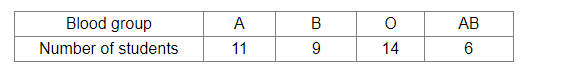# The following table shows the blood groups of 40 students of a class.

Question:

The following table shows the blood groups of 40 students of a class.One student of the class is chosen at random. What is the probability that the chosen student's blood group is
(i) O?
(ii) AB?

Solution:

Total number of students  = 4

(i) Number of students with blood group O = 14

Let E1 be the event that the selected student's blood group is O.

$\therefore$ Required probability $=P\left(E_{1}\right)=\frac{14}{40}=0.35$

(ii) Number of students with blood group AB = 6

Let E2 be the event that the selected student's blood group is AB.

$\therefore$ Required probability $=P\left(E_{2}\right)=\frac{6}{40}=0.15$